## Graeffe's Method

A Root-finding method which proceeds by multiplying a Polynomialbyand noting that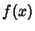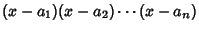(1)(2)

so the result is(3)

Repeat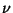times, then write this in the form(4)

where. Since the coefficients are given by Newton's Relations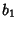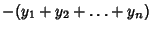(5)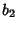(6)(7)

and since the squaring procedure has separated the roots, the first term is larger than rest. Therefore,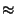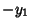(8)(9)(10)

giving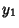(11)(12)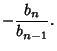(13)

Solving for the original roots gives(14)(15)(16)

This method works especially well if all roots are real.

References

von Kármán, T. and Biot, M. A. Squaring the Roots (Graeffe's Method).'' §5.8.c in Mathematical Methods in Engineering: An Introduction to the Mathematical Treatment of Engineering Problems. New York: McGraw-Hill, pp. 194-196, 1940.